# Class 8 RD Sharma Solutions – Chapter 17 Understanding Shapes Special Types Of Quadrilaterals – Exercise 17.1 | Set 2

### Question 16. The perimeter of a parallelogram is 150 cm. One of its sides is greater than the other by 25 cm. Find the length of the sides of the parallelogram.

Solution:

Given that,

Perimeter of the parallelogram = 150 cm

Let us assume that one of the sides as = ‘x’ cm

and other side as = (x + 25) cm

As we know that opposite sides of a parallelogram are parallel and equal.

Therefore, Perimeter = Sum of all sides

x + x + 25 + x + x + 25 = 150

4x + 50 = 150

4x = 150 – 50

x = 100/4 = 25

Hence, Sides of the parallelogram are (x) = 25 cm and (x+25) = 50 cm.

### Question 17. The shorter side of a parallelogram is 4.8 cm and the longer side is half as much again as the shorter side. Find the perimeter of the parallelogram.

Solution:

Given that,

Shorter side of the parallelogram = 4.8 cm

and longer side of the parallelogram = 4.8 + 4.8/2 = 4.8 + 2.4 = 7.2cm

As we know that opposite sides of a parallelogram are parallel and equal.

Therefore, Perimeter = Sum of all sides

Perimeter of the parallelogram = 4.8 + 7.2 + 4.8 + 7.2 = 24cm

Hence, Perimeter of the parallelogram is 24 cm.

### Question 18. Two adjacent angles of a parallelogram are (3x-4)o and (3x+10)°. Find the angles of the parallelogram.

Solution:

As we know that adjacent angles of a parallelogram are equal.

Therefore, (3x – 4)° + (3x + 10)° = 180°

3x° + 3xo – 4 + 10 = 180°

6x = 180° – 6°

x = 174°/6 = 29°

(3x – 4)° = 3×29 – 4 = 83°

(3x + 10)° = 3×29 + 10 = 97°

As we know that Sum of adjacent angles = 180°

Hence, each angle is 83°, 97°, 83°, 97°.

### Question 19. In a parallelogram ABCD, the diagonals bisect each other at O. If ∠ABC =30°, ∠BDC= 10° and ∠CAB =70°. Find:

∠DAB, ∠ADC, ∠BCD, ∠AOD, ∠DOC, ∠BOC, ∠AOB, ∠ACD, ∠CAB, ∠ADB, ∠ACB, ∠DBC, and ∠DBA.

Solution: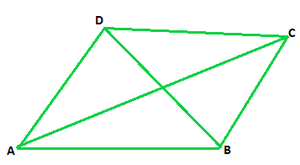Given that,

∠ABC = 30°,

∠ABC = ∠ADC = 30° (We know that measure of opposite angles are equal in a parallelogram)

∠BDC = 10°

∠CAB =70°

∠BDA = ∠ADB = ∠ADC – ∠BDC = 30° – 10° = 20° (From figure we concluded)

∠DAB = 180° – 30° = 150°

∠ADB = ∠DBC = 20° (alternate angles)

∠BCD = ∠DAB = 150° (we know, opposite angles are equal in a parallelogram)

∠DBA = ∠BDC = 10° (we know, Alternate interior angles are equal)

In ΔABC ∠CAB + ∠ABC + ∠BCA = 180° (since, sum of all angles of a triangle is 180°)

70° + 30° + ∠BCA = 180°

∠BCA = 180° – 100° = 80°

∠DAB = ∠DAC + ∠CAB = 70° + 80° = 150°

∠BCD = 150° (opposite angle of the parallelogram)

∠DCA = ∠CAB = 70°

In ΔDOC ∠BDC + ∠ACD + ∠DOC = 180° (since, sum of all angles of a triangle is 180°)

10° + 70° + ∠DOC = 180°

∠DOC = 180°- 80°

∠DOC = 100°

Therefore, ∠DOC = ∠AOB = 100° (Vertically opposite angles are equal)

∠DOC + ∠AOD = 180° [Linear pair]

100° + ∠AOD = 180°

∠AOD = 180°- 100°

∠AOD = 80°

Therefore, ∠AOD = ∠BOC = 80° (Vertically opposite angles are equal)

∠CAB = 70o

∠ABC + ∠BCD = 180° (In a parallelogram sum of adjacent angles is 180°)

30° + ∠ACB + ∠ACD = 180°

30° + ∠ACB + 70° = 180°

∠ACB = 180° – 100°

∠ACB = 80°

Hence ∠DAB = 150o, ∠ADC = 30°, ∠BCD = 150°, ∠AOD = 80°, ∠DOC = 100°, ∠BOC = 80°, ∠AOB = 100°, ∠ACD = 70°, ∠CAB = 70°, ∠ADB = 20°, ∠ACB = 80°, ∠DBC = 20°, and ∠DBA = 10°.

### Question 20. Find the angles marked with a question mark shown in Figure.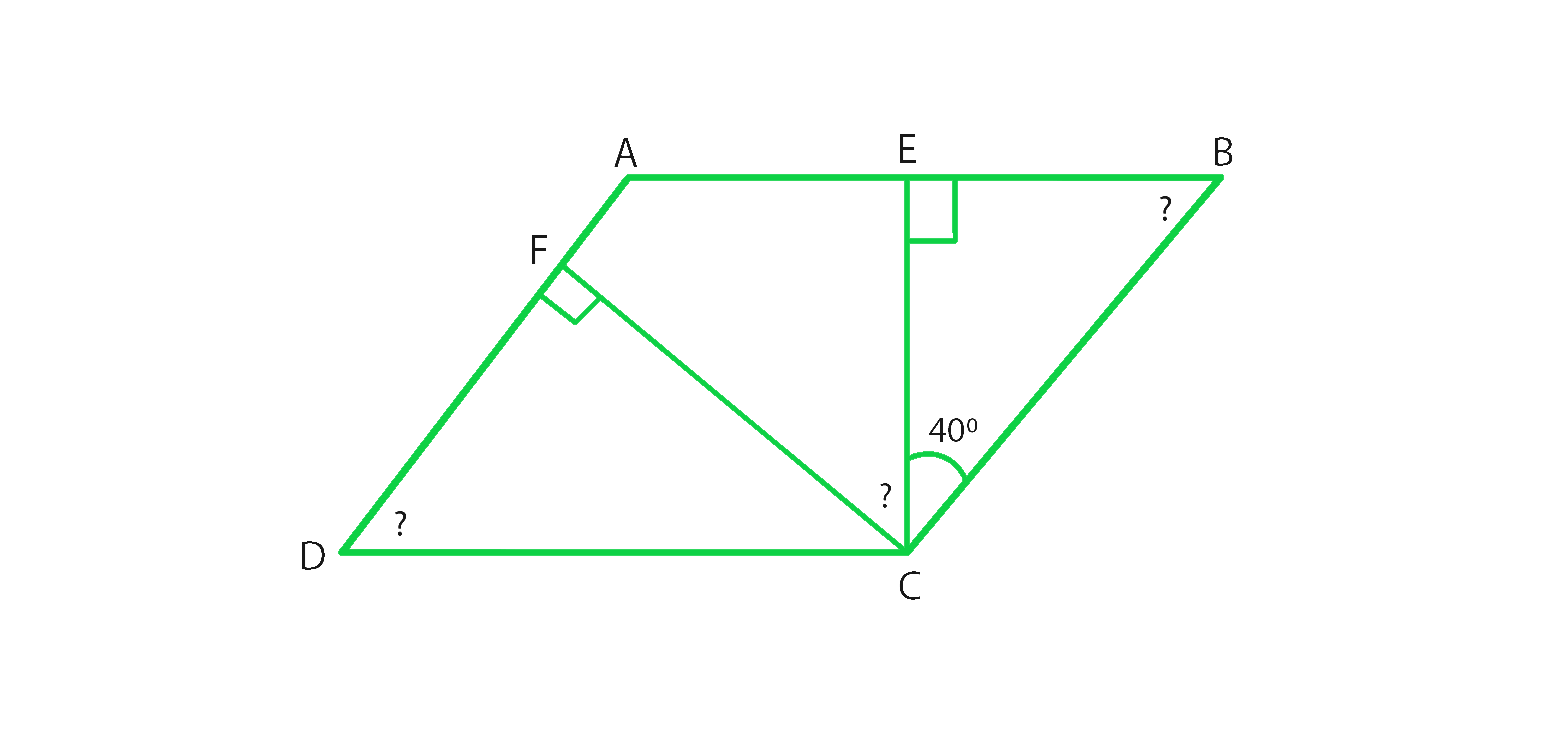Solution:

In ΔBEC ∠BEC + ∠ECB +∠CBE = 180° (Sum of angles of a triangle is 180°)

90° + 40° + ∠CBE = 180°

∠CBE = 180°-130°

∠CBE = 50°

∠CBE = ∠ADC = 50° (Opposite angles of a parallelogram are equal)

∠B = ∠D = 50° (opposite angles of a parallelogram are equal)

∠A + ∠B = 180° (Sum of adjacent angles of a triangle is 180°)

∠A + 50° = 180°

∠A = 180°-50°

therefore, ∠A = 130°

In ΔDFC ∠DFC + ∠FCD +∠CDF = 180° (Sum of angles of a triangle is 180°)

90° + ∠FCD + 50° = 180°

∠FCD = 180°-140°

∠FCD = 40°

∠A = ∠C = 130° (Opposite angles of a parallelogram are equal)

∠C = ∠FCE +∠BCE + ∠FCD

∠FCD + 40° + 40° = 130°

∠FCD = 130° – 80°

∠FCD = 50°

Hence ∠EBC = 50°, ∠ADC = 50° and ∠FCD = 50°.

### Question 21. The angle between the altitudes of a parallelogram, through the same vertex of an obtuse angle of the parallelogram, is 60°. Find the angles of the parallelogram.

Solution: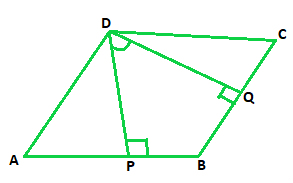Let us consider parallelogram ABCD, where DP⊥ AB and DQ ⊥ BC.

Given that ∠PDQ = 60°

In quadrilateral DPBQ ∠PDQ + ∠DPB + ∠B + ∠BQD = 360° (Sum of all the angles of a Quadrilateral is 360°)

60° + 90° + ∠B + 90° = 360°

∠B = 360° – 240°

∠B = 120°

∠B = ∠D = 120° (Opposite angles of parallelogram are equal)

∠B + ∠C = 180° (Sum of adjacent interior angles in a parallelogram is 180°)

120° + ∠C = 180°

∠C = 180° – 120° = 60°

∠A = ∠C = 60° (Opposite angles of parallelogram are equal)

Hence, Angles of a parallelogram are 60°, 120°, 60°, 120°

### Question 22. In Figure, ABCD and AEFG are parallelograms. If ∠C =55°, what is the measure of ∠F?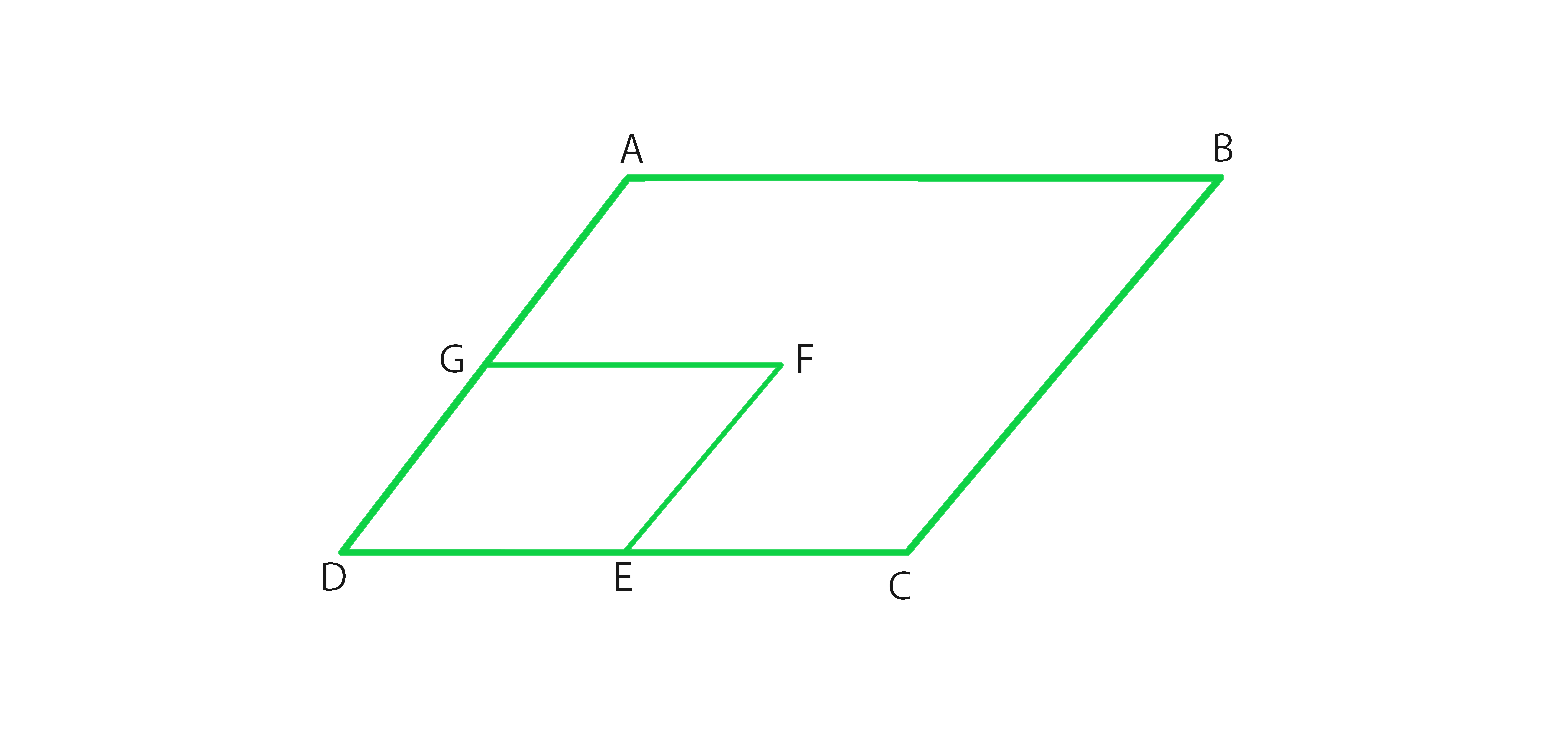Solution:

From figure, we conclude that,

In parallelogram ABCD ∠C = ∠A = 55° (In a parallelogram opposite angles are equal in a parallelogram)

In parallelogram AEFG ∠A = ∠F = 55° (In a parallelogram opposite angles are equal in a parallelogram)

Hence ∠F = 55°

### Question 23. In Figure, BDEF and DCEF are each a parallelogram. Is it true that BD = DC? Why or why not?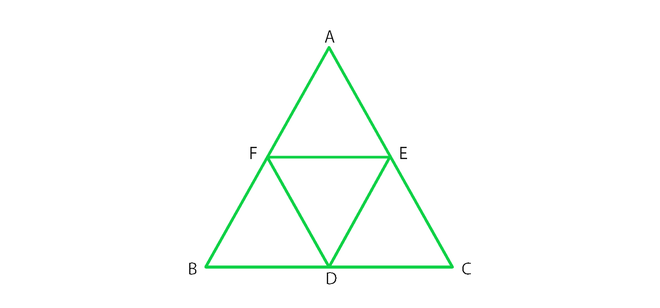Solution:

From figure, we conclude that,

In parallelogram BDEF BD = EF (In a parallelogram opposite sides are equal)

In parallelogram DCEF DC = EF (In a parallelogram opposite sides are equal)

Since, BD = EF = DC

Therefore, BD = DC

### Question 24. In Figure, suppose it is known that DE = DF. Then, is ΔABC isosceles? Why or why not?Solution:

From figure we conclude that,

In parallelogram BDEF BD = EF and BF = DE (opposite sides are equal in a parallelogram)

In parallelogram DCEF DC = EF and DF = CE (opposite sides are equal in a parallelogram)

In parallelogram AFDE AF = DE and DF = AE (opposite sides are equal in a parallelogram)

therefore, DE = AF = BF

similarly, DF = CE = AE

Given that, DE = DF

Since, DF = DF

AF + BF = CE + AE

AB = AC

Hence, ΔABC is an isosceles triangle.

### Question 25. Diagonals of parallelogram ABCD intersect at O as shown in Figure. XY contains O, and X, Y are points on opposite sides of the parallelogram. Give reasons for each of the following:

(i) OB = OD

(ii) ∠OBY = ∠ODX

(iii) ∠BOY = ∠DOX

(iv) ΔBOY = ΔDOX

Now, state if XY is bisected at O.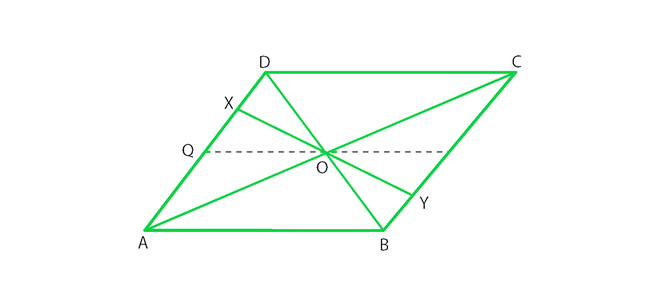Solution:

(i) OB = OD

OB = OD. Since diagonals bisect each other in a parallelogram.

(ii) ∠OBY =∠ODX

∠OBY =∠ODX. Since alternate interior angles are equal in a parallelogram.

(iii) ∠BOY= ∠DOX

∠BOY= ∠DOX. Since vertical opposite angles are equal in a parallelogram.

(iv) ΔBOY ≅ ΔDOX

ΔBOY and ΔDOX. Since OB = OD, where diagonals bisect each other in a parallelogram.

∠OBY =∠ODX (Alternate interior angles are equal)

∠BOY= ∠DOX (Vertically opposite angles are equal)

ΔBOY ≅ΔDOX (by ASA congruence rule)

OX = OY (Corresponding parts of congruent triangles)

Hence XY is bisected at O.

### Question 26. In Fig., ABCD is a parallelogram, CE bisects ∠C and AF bisects ∠A. In each of the following, if the statement is true, give a reason for the same:

(i) ∠A = ∠C

(ii) ∠FAB = ½ ∠A

(iii) ∠DCE = ½ ∠C

(iv) ∠CEB = ∠FAB

(v) CE ∥ AF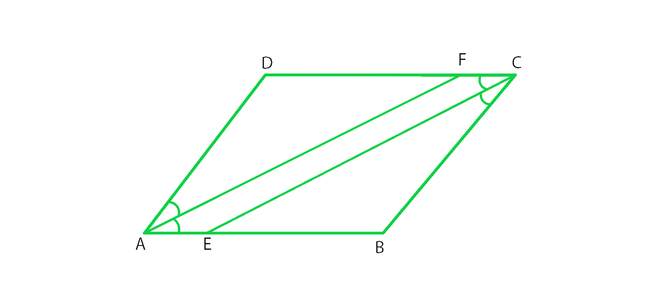Solution:

(i) ∠A = ∠C

True, Since ∠A =∠C = 55° [opposite angles are equal in a parallelogram]

(ii) ∠FAB = ½ ∠A

True, Since AF is the angle bisector of ∠A.

(iii) ∠DCE= ½ ∠C

True, Since CE is the angle bisector of angle ∠C.

(iv) ∠CEB= ∠FAB

True, Since ∠DCE = ∠FAB (opposite angles are equal in a parallelogram).

∠CEB = ∠DCE (alternate angles)

½ ∠C = ½ ∠A [AF and CE are angle bisectors]

(v) CE || AF

True, since one pair of opposite angles are equal, therefore quad. AEFC is a parallelogram.

### Question 27. Diagonals of a parallelogram ABCD intersect at O. AL and CM are drawn perpendiculars to BD such that L and M lie on BD. Is AL = CM? Why or why not?

Solution: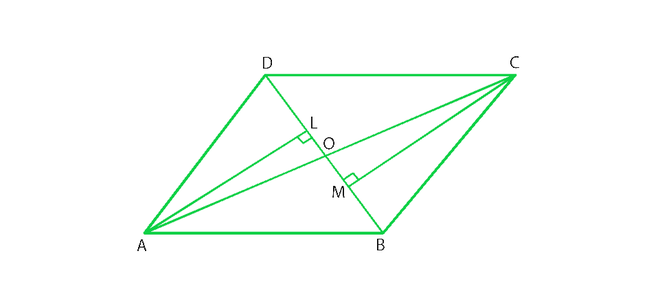Given that,

AL and CM are perpendiculars on diagonal BD.

In ΔAOL and ΔCOM,

∠AOL = ∠COM (vertically opposite angle) ———–(i)

∠ALO = ∠CMO = 90° (each right angle) ——–(ii)

By using angle sum property,

∠AOL + ∠ALO + ∠LAO = 180° ———(iii)

∠COM + ∠CMO + ∠OCM = 180° ———- (iv)

From (iii) and (iv)

∠AOL + ∠ALO + ∠LAO = ∠COM + ∠CMO + ∠OCM

∠LAO = ∠OCM (from (i) and (ii))

In ΔAOL and ΔCOM

∠ALO = ∠CMO (each right angle)

AO = OC (diagonals of a parallelogram bisect each other)

∠LAO = ∠OCM (proved)

therefore, ΔAOL is congruent to ΔCOM

Hence AL = CM (Corresponding parts of congruent triangles)

### Question 28. Points E and F lie on diagonals AC of a parallelogram ABCD such that AE = CF. what type of quadrilateral is BFDE?

Solution: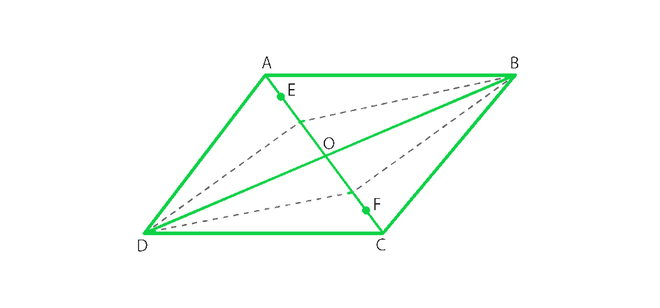From figure, we conclude that,

In parallelogram ABCD,

AO = OC ———- (i) (Diagonals of a parallelogram bisect each other)

AE = CF ———-(ii) Given

On subtracting (ii) from (i)

AO – AE = OC – CF

EO = OF ——-(iii)

In ΔDOE and ΔBOF,

EO = OF (proved)

DO = OB (Diagonals of a parallelogram bisect each other)

∠DOE = ∠BOF (vertically opposite angles are equal in a parallelogram)

By SAS congruence ΔDOE ≅ ΔBOF

therefore, DE = BF (Corresponding parts of congruent triangles)

In ΔBOE and ΔDOF,

EO = OF (proved)

DO = OB (diagonals of a parallelogram bisect each other)

∠DOF = ∠BOE (vertically opposite angles are equal in a parallelogram)

By SAS congruence ΔDOE ≅ ΔBOF

Hence, DF = BE (Corresponding parts of congruent triangles).

Hence, BFDE is a parallelogram, since one pair of opposite sides are equal and parallel.

### Question 29. In a parallelogram ABCD, AB = 10cm, AD = 6 cm. The bisector of ∠A meets DC in E, AE and BC produced meet at F. Find the length CF.

Solution: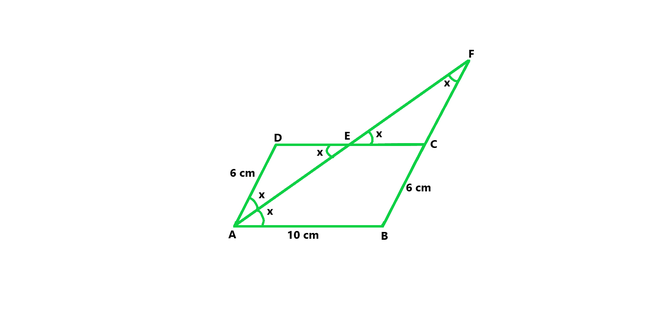From figure we concluded that,

In a parallelogram ABCD,

Given, AB = 10 cm, AD = 6 cm

CD = AB = 10 cm and AD = BC = 6 cm (In a parallelogram opposite sides are equal)

AE is the bisector of ∠DAE = ∠BAE = x

∠BAE = ∠AED = x (alternate angles are equal)

ΔADE is an isosceles triangle. Since opposite angles in ΔADE are equal.

AD = DE = 6cm (opposite sides are equal)

CD = DE + EC

EC = CD – DE = 10 – 6 = 4cm

∠DEA = ∠CEF = x (vertically opposite angle are equal)

∠EAD = ∠EFC = x (alternate angles are equal)

ΔEFC is an isosceles triangle. Since opposite angles in ΔEFC are equal.

CF = CE = 4cm (opposite side are equal to angles)

Hence CF = 4cm.

Whether you're preparing for your first job interview or aiming to upskill in this ever-evolving tech landscape, GeeksforGeeks Courses are your key to success. We provide top-quality content at affordable prices, all geared towards accelerating your growth in a time-bound manner. Join the millions we've already empowered, and we're here to do the same for you. Don't miss out - check it out now!

Previous
Next